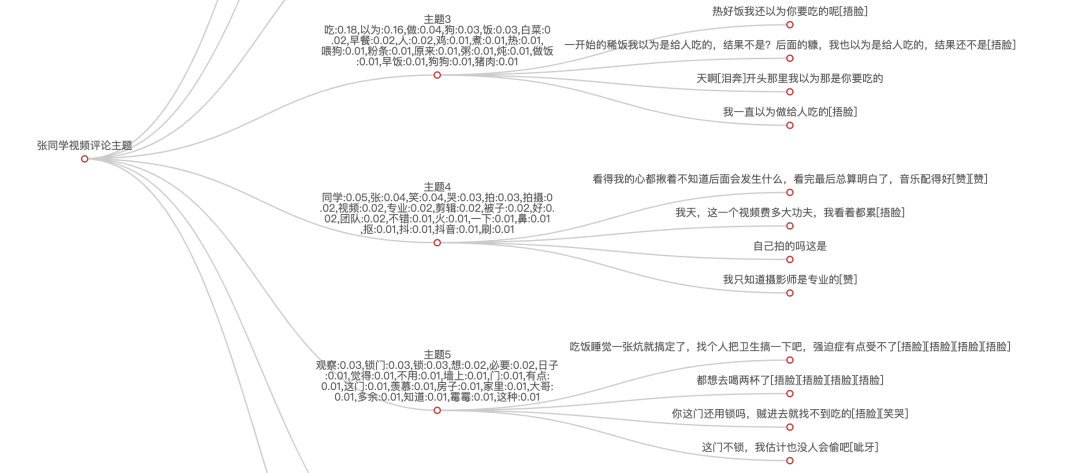﻿ Python 抖音评论数据抓取分析_python_脚本之家
python# Python 抖音评论数据抓取分析## 1. 抓取数据• 有时候接口可能返回空数据，因此需要多试几次，一般过了人工滑动验证后的接口基本可用
• 不同页面之间的数据可能会重复，所以需要跳页请求

## 2. EDA

11.17 号的视频有 12w 条评论，我只抓取了 1w 多条。text列是评论。

```# eda
profile = ProfileReport(df, title='张同学抖音评论数据', explorative=True)
profile
```## 3. LDA

LDA算法的核心思想有两点：

• 具有一定相似性的文本会聚合在一起，形成一个主题。每个主题包含生成该主题需要的词，以及这些词的概率分布。以此可以人为推断出主题的类别。
• 每篇文章会它有在所有主题下的概率分布，以此可以推断出文章属于哪个主题。

### 3.1 分词、去停用词

```# 分词

emoji = {'可怜', '发呆', '晕', '灵机一动', '击掌', '送心', '泣不成声', '哈欠', '舔屏', '偷笑', '愉快', '再见', '666', '熊吉', '尬笑', '吐舌', '撇嘴', '看', '绿帽子', '捂脸', '呆无辜', '强壮', '震惊', '阴险', '绝', '给力', '打脸', '咖啡', '衰', '一起加油', '酷拽', '流泪', '黑脸', '爱心', '笑哭', '机智', '困', '微笑袋鼠', '强', '闭嘴', '来看我', '色', '憨笑', '不失礼貌的微笑', '红脸', '抠鼻', '调皮', '紫薇别走', '赞', '比心', '悠闲', '玫瑰', '抱拳', '小鼓掌', '握手', '奸笑', '害羞', '快哭了', '嘘', '惊讶', '猪头', '吐', '暗中观察', '不看', '啤酒', '呲牙', '发怒', '绝望的凝视', '大笑', '吐血', '坏笑', '凝视', '可爱', '拥抱', '擦汗', '鼓掌', '胜利', '感谢', '思考', '微笑', '疑问', '我想静静', '灵光一闪', '白眼', '泪奔', '耶'}
stopwords = [line.strip() for line in open('stop_words.txt', encoding='UTF-8').readlines()]

def fen_ci(x):
res = []
for x in jieba.cut(x):
if x in stopwords or x in emoji or x in ['[', ']']:
continue
res.append(x)
return ' '.join(res)

df['text_wd'] = df['text'].apply(fen_ci)
```

### 3.2 调用LDA

```from sklearn.feature_extraction.text import CountVectorizer
from sklearn.decomposition import LatentDirichletAllocation
import numpy as np

def run_lda(corpus, k):
cntvec = CountVectorizer(min_df=2, token_pattern='\w+')
cnttf = cntvec.fit_transform(corpus)

lda = LatentDirichletAllocation(n_components=k)
docres = lda.fit_transform(cnttf)

return cntvec, cnttf, docres, lda

cntvec, cnttf, docres, lda = run_lda(df['text_wd'].values, 8)
```### 主题的词分布